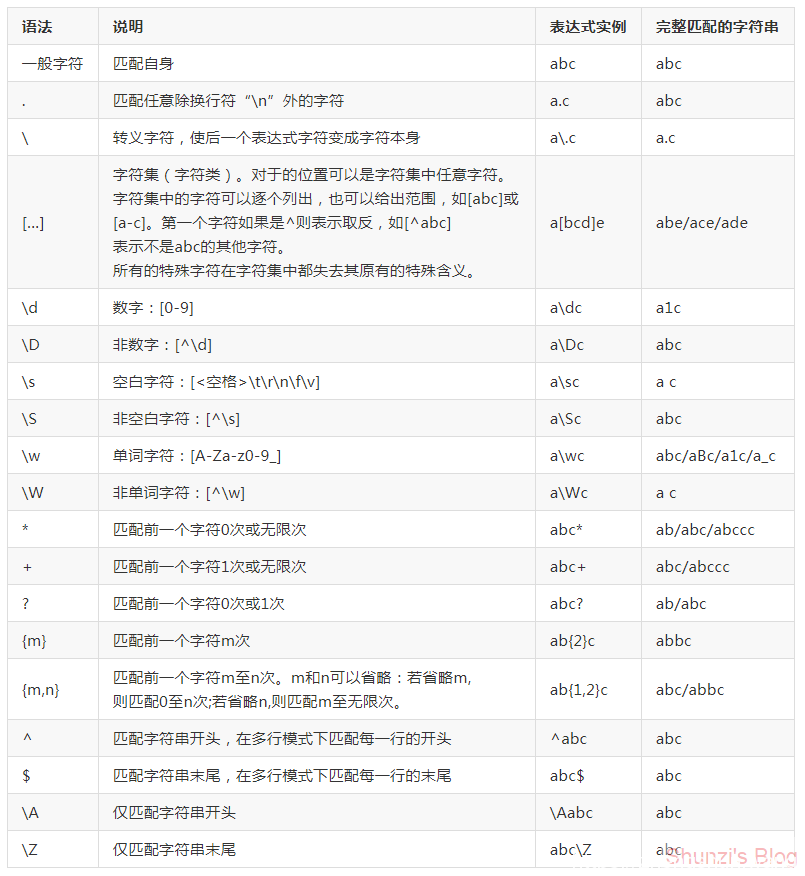# Oracle正则表达式

• A+

### 正则表达式具体描述| 竖杠代表作有表达式任意匹配一个，他总是先尝试左边的表达式，一旦成功匹配则跳过匹配右边的表达式，如果|没有被包括在()中，则它的范围是整个正则表达式。 (...) 被括起来的表达式将作为分组，从表达式左边开始没遇到一个分组的做客户'('，编号+1.另外，分组表达式作为一个整体，可以后接数量词，表达式中的|仅在改组中有效。

### 正则字符簇

• [[:alpha:]] 匹配任意字母
• [[:digit:]] 匹配任意数字
• [[:alnum:]] 匹配任意字母和数字
• [[:space:]] 匹配任意白字符
• [[:upper:]] 匹配任意大写字母
• [[:lower:]] 匹配任意小写字母
• [[unct:]] 匹配任意标点符号
• [[:xdigit:]] 匹配任意16进制的数字，相当于[0-9a-fA-F]

### Oracle正则表达式函数

1. regexp_substr 使用方法：regexp_substr(source_string,pattern[,position[,occurrence[,match_parameter]]])

• source_string：源串，可以是常量，也可以是某个值类型为串的列。
• pattern:正则表达式
• position：从源串开始搜索的位置。默认为1。
• occurrence：指定源串中的第几次出现。默认值1.
• match_parameter：文本量，进一步订制搜索，取值如下：
• 'i' 用于不区分大小写的匹配。
• 'c' 用于区分大小写的匹配。
• 'n' 允许将句点“.”作为通配符来匹配换行符。如果省略改参数，句点将不匹配换行符。
• 'm' 将源串视为多行。即将“^”和“$”分别看做源串中任意位置任意行的开始和结束，而不是看作整个源串的开始或结束。如果省略该参数，源串将被看作一行来处理。 • 如果取值不属于上述中的某个，将会报错。如果指定了多个互相矛盾的值，将使用最后一个值。如'ic'会被当做'c'处理。 • 省略该参数时：默认区分大小写、句点不匹配换行符、源串被看作一行。 2. regexp_instr 使用方法：regexp_instr(source_string,pattern[,position[,occurrence[,return_option[,match_parameter]]]]) • return_option：为0时，返回第一个字符出现的位置，与instr作用相同。为1时，返回所搜索字符出现以后下一个字符的位置。默认为0. 3. regexp_like 使用方法：regexp_like(source_string,pattern[match_parameter]) • 该函数可以使用前面介绍的所有搜索功能作为REGEXP_LIKE搜索的一部分，可以是非常复杂的搜索变得简单。 4. regexp_replace 使用方法：regexp_replace(source_string,pattern[,replace_string[,position[,occurrence[,match_parameter]]]]) • replace_string表示用什么来替换source_string中与pattern匹配的部分。 • 如果不指定replace_string，会将搜索到的值删除。 • occurrence为非负整数，0表示所有匹配项都被替换，为正数时替换第n次匹配。 • 其他参数在前面都已经介绍过了。 5. regexp_count 使用方法：regexp_count(source_char,pattern[,position[,match_param]]) • regexp_count返回pattern在source_char串中出现的次数。如果未找到匹配，函数返回0。 • metch_param参数，相对于前面介绍的match_parameter参数多一个取值“x”。 • 'x'：忽略空格字符。默认情况下，空格与自身想匹配。 • metch_param如果指定了多个互相矛盾的值，将使用最后一个值。 ### 模拟测试例子 create table test(mc varchar2(60)); insert into test values('112233445566778899'); insert into test values('22113344 5566778899'); insert into test values('33112244 5566778899'); insert into test values('44112233 5566 778899'); insert into test values('5511 2233 4466778899'); insert into test values('661122334455778899'); insert into test values('771122334455668899'); insert into test values('881122334455667799'); insert into test values('991122334455667788'); insert into test values('aabbccddee'); insert into test values('bbaaaccddee'); insert into test values('ccabbddee'); insert into test values('ddaabbccee'); insert into test values('eeaabbccdd'); insert into test values('ab123'); insert into test values('123xy'); insert into test values('007ab'); insert into test values('abcxy'); insert into test values('The final test is is is how to find duplicate words.'); commit; REGEXP_LIKE select * from test where regexp_like(mc,'^a{1,3}'); select * from test where regexp_like(mc,'a{1,3}'); select * from test where regexp_like(mc,'^a.*e$');
select * from test where regexp_like(mc,'^[[:lower:]]|[[:digit:]]');
select * from test where regexp_like(mc,'^[[:lower:]]');
Select mc FROM test Where REGEXP_LIKE(mc,'[^[:digit:]]');
Select mc FROM test Where REGEXP_LIKE(mc,'^[^[:digit:]]');

REGEXP_INSTR

Select REGEXP_INSTR(mc,'[[:digit:]]$') from test; Select REGEXP_INSTR(mc,'[[:digit:]]+$') from test;
Select REGEXP_INSTR('The price is $400.','\$[[:digit:]]+') FROM DUAL;
Select REGEXP_INSTR('onetwothree','[^[[:lower:]]]') FROM DUAL;
Select REGEXP_INSTR(',,,,,','[^,]*') FROM DUAL;
Select REGEXP_INSTR(',,,,,','[^,]') FROM DUAL;

REGEXP_SUBSTR

SELECT REGEXP_SUBSTR(mc,'[a-z]+') FROM test;
SELECT REGEXP_SUBSTR(mc,'[0-9]+') FROM test;
SELECT REGEXP_SUBSTR('aababcde','^a.*b') FROM DUAL;

REGEXP_REPLACE

Select REGEXP_REPLACE('Joe Smith','( ){2,}', ',') AS RX_REPLACE FROM dual;
Select REGEXP_REPLACE('aa bb cc','(.*) (.*) (.*)', '\3, \2, \1') FROM dual;

REGEXP_COUNT

SELECT REGEXP_COUNT('+CRMADG/CRMASTDY/datafile/system.299.846863455', '/')   FROM DUAL;

### 总结